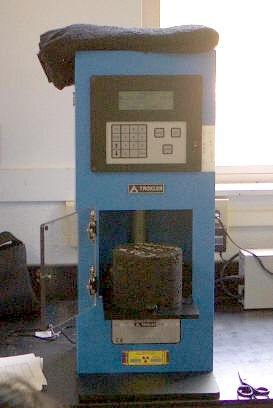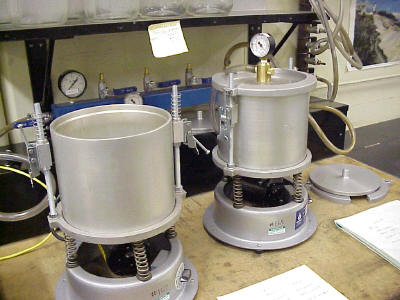# Mixture Characterization Tests

Mixture characterization tests are used to describe fundamental mixture parameters such as density and asphalt binder content.  The three primary mixture characterization tests discussed here are:

### Bulk Specific Gravity

Bulk specific gravity is essentially the density of a compacted (laboratory or field) HMA specimen.  The bulk specific gravity is a critical HMA characteristic because it is used to calculate most other HMA parameters including air voids, VMA, and TMD.  This reliance on bulk specific gravity is because mix design is based on volume, which is indirectly determined using mass and specific gravity.  Bulk specific gravity is calculated as:

There are several different ways to measure bulk specific gravity, all of which use slightly different ways to determine specimen volume:

1. Water displacement methods.  These methods, based on Archimedes Principle, calculate specimen volume by weighing the specimen (1) in a water bath and (2) out of the water bath.  The difference in weights can then be used to calculate the weight of water displaced, which can be converted to a volume using the specific gravity of water.
• Saturated Surface Dry (SSD).  The most common method, calculates the specimen volume by subtracting the mass of the specimen in water from the mass of a saturated surface dry (SSD) specimen.  SSD is defined as the specimen condition when the internal air voids are filled with water and the surface (including air voids connected to the surface) is dry.  This SSD condition allows for internal air voids to be counted as part of the specimen volume and is achieved by soaking the specimen in a water bath for 4 minutes then removing it and quickly blotting it dry with a damp towel.  One critical problem with this method is that if a specimen’s air voids are high, and thus potentially interconnected (for dense-graded HMA this occurs at about 8 to 10 percent air voids), water quickly drains out of them as the specimen is removed from its water bath, which results in an erroneously low volume measurement and thus an erroneously high bulk specific gravity.
• Paraffin.  This method determines volume similarly to the water displacement method but uses a melted paraffin wax instead of water to fill a specimen’s internal air voids (see Figure 1).  Therefore, after the wax sets there is no possibility of it draining out and, theoretically, a more accurate volume can be calculated.  In practice, the paraffin is difficult to correctly apply and test results are somewhat inconsistent.Figure 1. Paraffin Coated Sample
• Parafilm.  This method wraps the specimen in a thin paraffin film (see Figure 2) and then weighs the specimen in and out of water.  Since the specimen is completely wrapped when it is submerged, no water can get into it and a more accurate volume measurement is theoretically possible.  However, in practice the paraffin film application is quite difficult and test results are inconsistent.Figure 2. Parafilm Application
• CoreLok.  This method calculates specimen volume like the parafilm method but uses a vacuum chamber (see Figure 3) to shrink-wrap the specimen in a high-quality plastic bag (see Figure 4) rather than cover it in a paraffin film.  This method has shown some promise in both accuracy and precision.Figure 3. CoreLok Vacuum ChamberFigure 4. CoreLok Specimen
• Dimensional.  This method, the simplest, calculates the volume based on height and diameter/width measurements.  Although it avoids problems associated with the SSD condition, it is often inaccurate because it assumes a perfectly smooth surface thereby ignoring surface irregularities (i.e., the rough surface texture of a typical specimen).Figure 5.Gamma Ray Device
• Gamma ray.  The gamma ray method is based on the scattering and absorption properties of gamma rays with matter. When a gamma ray source of primary energy in the Compton range is placed near a material, and an energy selective gamma ray detector is used for gamma ray counting, the scattered and unscattered gamma rays with energies in the Compton range can be counted exclusively. With proper calibration, the gamma ray count is directly converted to the density or bulk specific gravity of the material (Troxler, 2001).  Figure 5 shows the Troxler device.

The standard bulk specific gravity test is:

• AASHTO T 166: Bulk Specific Gravity of Compacted Bituminous Mixtures Using Saturated Surface-Dry Specimens (this is the SSD water displacement method discussed previously)

### Theoretical Maximum Specific Gravity

The theoretical maximum specific gravity (often referred to as theoretical maximum density and thus abbreviated TMD) is the HMA density excluding air voids.  Thus, theoretically, if all the air voids were eliminated from an HMA sample, the combined density of the remaining aggregate and asphalt binder would be the TMD – often referred to as Rice density after its inventor.  TMD is a critical HMA characteristic because it is used to calculate percent air voids in compacted HMA and provide target values for HMA compaction.

TMD is determined by taking a sample of oven-dry HMA in loose condition (versus compacted condition), weighing it and then completely submerging it in a 25°C water bath.  A vacuum is then applied for 15 minutes (see Figure 6) to remove any entrapped air.  The sample volume is then calculated by subtracting its mass in water from its dry mass.  The formula for calculating TMD is:where: TMD = theoretical maximum density A = mass of oven dry sample in air in grams C = mass of water displaced by sample at 25°C in gramsFigure 6. Containers Used to Agitate and Draw a Vacuum on Submerged TMD Samples

The standard TMD test is:

AASHTO T 209 and ASTM D 2041: Theoretical Maximum Specific Gravity and Density of Bituminous Paving Mixtures

Footnotes    (↵ returns to text)
1. Troxler Electronic Laboratories, Inc. (Troxler).  (March 2001).  Measuring Bulk Specific Gravity of Compacted Specimens Using The Troxler Model 3660 CoreReader.  Web page on the Troxler web site.  Troxler Electronic Laboratories, Inc.  Research Triangle Park, NC.  Accessed 1 July 2002.